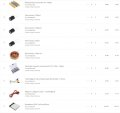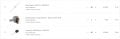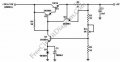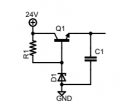# Updating my Equipment + Func Generator Project + 24Vdc Power Supply Project

#### babaliaris

Joined Nov 19, 2019
158Function Generator Project:
I thought of using my phone as a frequency low amplitude generator (using my phone's headphone jack 3.5mm as output).
Then using a Commoner Emitter Amplifier (I hope I will know how to design one by then) I will amplify it.
This is probably a simple function generator to have something to play with in my small home labPower Supply 24DC:
Using the 230V/24V 10VA 400mA transformer + 4 rectifier diodes + a capacitor + voltage regulation, I can make a simple power supply.
The voltage regulation will be with a Zener diode probably. Maybe I can make it Zener + NPN (but I need a separate power supply for the transistor).

Any tips? I think these two projects will be amazing for the first application of my knowledge.
Of course, I will post my projects here, and simulate them in LTSpice before trying to attempt to build them and power them up.

Last edited by a moderator:

#### dl324

Joined Mar 30, 2015
15,471
Any tips?
That's going to be a pretty wimpy power supply. Do you know how much of the 400mA you'll lose from rectification/filtering?

Since you're still learning how to design circuits, I'd suggest that you use this classical discrete design and try to understand how every component and value was selected.The voltage regulation will be with a Zener diode probably. Maybe I can make it Zener + NPN (but I need a separate power supply for the transistor).
Do you realize that this will make a pretty crappy power supply? Assuming you mean something like this:Last edited:

#### babaliaris

Joined Nov 19, 2019
158
Do you realize that this will make a pretty crappy power supply? Assuming you mean something like this:
View attachment 282635
If we assume a value for Vb, let's say this Zener diode is 20V. Then

$$V_{b} - V_{e} \approx 0.7 <=> V_{e} \approx V_{b} - 0.7 = 20 - 0.7 = 19.3V$$

$$V_{c} - V_{e} = 24 - 19.3 = 4.7V$$ We are close to saturation.

In other words, Ic is big and most of the power gets dissipated in the transistor.

Since you're still learning how to design circuits, I'd suggest that you use this classical discrete design and try to understand how every component and value was selected.
View attachment 282632
In this circuit, you are trying to regulate 10 - 15Vdc to 5Vdc. Through Q2->Q3->Q4 you reduce the voltage by 0,7V each time, so at the node between R2 AND R3, you have reduced it 3 times 0.7V. Afterward, you divide this voltage through the voltage divider $$\frac{R5}{(R3+R4) + R5}$$ and you drop that another 0.7V in Q1 (Don't know why). I'm not sure how you get 5V at this end unless I do the complete theoretical analysis of the circuit.

But I think the idea here is to divide the current through all these transistors in order to regulate the voltage with the lowest power dissipation, right?

Before we continue, let's make sure that I understand so far.

#### Jony130

Joined Feb 17, 2009
5,435
Why don't you use a voltage regulator? LM78XX or adjustable version LM317?
Also are you aware that the voltage after rectification (plus smoothing capacitor) will be equal to about 24V * 1.41 - 1.2V = 33V and without a load even higher (around 38V)?

And the output voltage of a discrete voltage regulator is equal to :

$$V_O = \left(V_{D1} + V_{BE1}\right) * \left( 1 + \frac{R3 + R4}{R_5} \right)$$

So, for the values of the resistors given on the schematics the output voltage can be adjustable from around 1.2V * (1 + 1/1) = 2.4V to 1.3V*(1 + 6/1) = 8.4V.
How this circuit work you can find here:
https://tinyurl.com/2f3u88t9

#### dl324

Joined Mar 30, 2015
15,471
In other words, Ic is big and most of the power gets dissipated in the transistor.
The problem is that the output voltage will depend on the BE voltage drop in the pass transistor which will vary with load. That will give you pretty crappy load regulation.

•babaliaris

#### babaliaris

Joined Nov 19, 2019
158
Why don't you use a voltage regulator? LM78XX or adjustable version LM317?
Also are you aware that the voltage after rectification (plus smoothing capacitor) will be equal to about 24V * 1.41 - 1.2V = 33V and without a load even higher (around 38V)?

And the output voltage of a discrete voltage regulator is equal to :

$$V_O = \left(V_{D1} + V_{BE1}\right) * \left( 1 + \frac{R3 + R4}{R_5} \right)$$

So, for the values of the resistors given on the schematics the output voltage can be adjustable from around 1.2V * (1 + 1/1) = 2.4V to 1.3V*(1 + 6/1) = 8.4V.
How this circuit work you can find here:
https://tinyurl.com/2f3u88t9
I thought things were easier... How will I learn then?

#### Jony130

Joined Feb 17, 2009
5,435

#### dl324

Joined Mar 30, 2015
15,471
I thought things were easier... How will I learn then?
You'll learn more if you try to use your existing knowledge to determine how the voltage regulator circuit I posted works before looking for explanations of how it works. Identify the circuit blocks, then try to understand why specific components and values were chosen.

For instance, why was a 1N4001 diode used? Is a rectifier diode required? What is the purpose of C1? Does it need to be 1000uF?

When analyzing the voltage divider, just pick a resistance between 0-5k for the pot. Then change the value to see how the circuit behaves.

This circuit was probably the 3rd voltage regulator we studied when I was in college. The circuit you proposed was what we analyzed after using a voltage divider.

EDIT: I tracked down the page where the schematic I posted was from (I just pulled a picture of a reasonable looking circuit). Discrete Voltage Regulator – Electronic Circuit Diagram (freecircuitdiagram.com)

Apparently it was derived from a circuit that used a zener diode instead of the rectifier diode. Unfortunately, the author didn't give the original author credit, so s/he remains unknown. It's a classical design found in many text books.

Last edited:
•babaliaris

#### babaliaris

Joined Nov 19, 2019
158
This is a piece of really good advice, didn't think of it. I will use it in every circuit I want to learn how it works.

By the way, if a circuit is big, is it a good idea to try and divide it into smaller circuits and try to analyze each on of the separately?

#### dl324

Joined Mar 30, 2015
15,471
This is a piece of really good advice, didn't think of it. I will use it in every circuit I want to learn how it works.
That's a technique Bill Hewlett was said to have used.

By the way, if a circuit is big, is it a good idea to try and divide it into smaller circuits and try to analyze each on of the separately?
Divide and conquer is usually a good circuit analysis technique.

Don't know if you saw the link to the original schematic I added to my previous post.

•babaliaris